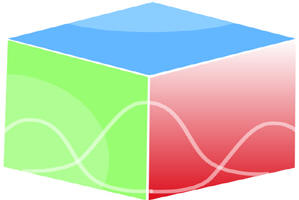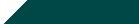``Home| Journals | Statistics Online Expert | About Us | Contact UsUntitled Document

[

Sasakian Metric as Yamabe Soliton

Ankita Rai1*, Dhruwa Narain2

Department of Mathematics and Statistics, D.D.U. Gorakhpur University, Gorakhpur- 273009

Research Article

Abstract               In this paper we study Sasakian manifold whose metric is as Yamabe soliton and obtain some result.

Key words: Sasakian metric, Yamabe soliton, cconstant scalar cuvature, infinitesimal automorphism.

Mathematical Subject Classification 2010: 53C25, 53C21, 53C44.

INTRODUCTION

For a smooth Riemannian manifold , the evolution of the metric  in the  to  through the equation:

( denotes the scalar curvature of ), is known as Yamabe flow, and was introduced by Hamilton . The significance of Yamabe flow lies in the fact that is a natural geometry deformation to metric of constant scalar curvature. One notes that Yamabe flow corresponds to the fact diffusion case of the porous medium equation ( the plasma equation) in Mathematical physics. Just as Ricci soliton is a special solution of the Ricci flow, A Yamabe soliton is a special solution of Yamabe flow that moves by one parameter family of diffeomorphism  generated by a fixed (time independent) vector field V on M, and homotheties, i.e. . Equivalently, a Yamabe soliton is defined on a Riemannian manifold  (suppressing the subscript 0 in ) by a vector field  satisfying;

(1)

where  denotes the derivative operator along ,  is the scalar curvature (not necessarily constant) of , and the constant  (see Chow et al. ). Recently, Sharma and Ghosh  studied a non-trivial Ricci soliton as a Sasakian manifold  and show  is a homothetic to the standard Sasakian metric on the Heisenberg nill3. The purpose of this paper is to study a Yamabe soliton as a Sasakian metric and prove the following results,

Theorem: If a Sasakian metric on a manifold  is a Yamab soliton, then it has constant scalar curvature, and the flow vector field is killing. Further  is also an infinitesimal automorphism of the contact metric structure on .

As indicated in , the scalar curvature of a Yamabe soliton on a compact manifold is constant. Theorem 1 replaces the compactness with Sasakian condition, and provides more in the conclusion. At this point, we would like to point out that eq. (1) define a conformal vector field  with conformal scale function , and that a Sasakian manifold with a non-isometric conformal vector field. We also point out that we have assumed the initial metric  to be Sasakian, however the subsequent metrics  along the Yamabe flow need not be Sasakian.

Preliminaries

A -dimensional smooth manifold  is said to be contact manifold if it carries a global 1-form  such that  everywhere on . Given a contact 1-form  there exists a unique vector field  such that  and  Polarizing  on the contact subbundle , one obtain a Riemannian metric  and a -tensor field  such that,

,                                                                                      (2)

is called a contact metric associated with . A vector field  on a contact metric manifold is said to be an infinitesimal contact transformation (see Tanno ) if  for some smooth function , and is said to be an infinitesimal automorphism of a contact metric structure if it is leaves all the structure tensors  and  invarriant (see Tanno ).

A contact metric is said to be K-contact if  is Killing with respect to . A contact metric manifold  is Sasakian if the cone manifold  is Kahler, Sasakian metric are K-contact and K-contact 3-dimensional metrics are Sasakian. For Sasakian manifold,

(3)

(4)

,                                                                                                                                                          (5)

where and  denotes respectively, the Riemannian connection and curvature tensor and the -tensor metrically equivalent to the Ricci tensor of .

Let us now briefly review conformal vector fields. A vector field on an -dimensional Riemannian manifold  is said to be conformal if,

(6)

for a smooth function  on M. A conformal vector satisfies,

(7)

(8)

where  is the gradiant operator and  is the Laplacian operator of . For detail we refer to . Further there is a lemma (we refer to Sharma ) which is, for a Sasakian manifold, (a).  and (b). .

SASAKIAN METRIC AS YAMABE SOLITON

The Ricci tensor S of a Sasakian metric is given by,

(9)

where  and β are constant. As  is a conformal vector field with , equ. (7) and (8) can be written as,

(10)

(11)

taking Lie-derivative of (9) along V and using equ. (1), (10) and (11) we obtains,

(12)

As  is killing, we have  Differentiating it along an arbitrary vector field X and using (2) gives,

Substituting  in place of  in (12) and using the above equation and lemma provides the equation,

(13)

Substituting  for  in the above equation, using (2) and lemma, we immediately get,

(14)

In view of the equation (14) and (13) shows that,

(15)

From (14) and (15), equation (13) becomes,

(16)

At this point we assume that  to be local orthonormal frame on M. Using (3.8) we compute,

And then using (2.1), (2.2), skew-symmetry of  and the second equation of (2.3) we obtain,

Where  is the summer over. The use of (16) in the right hand side of the foregoing equation show that , using this in (9) immediately yield  which gives  Hence we conclude that  is constant. From (14) we find that  Thus from (1) we find that  i.e  is killing. From equation (13) we conclude that  As  is killing, we also conclude that  Finally, taking Lie-derivative of first equation in (2) along  and noting that Lie-derivative commutes with exterior derivative, we conclude that  Thus,  is an infinitesimal automorphism of the contact metric structure of .

Theorem: If a Sasakian metric on a manifold  is a Yamabe soliton, then it has constant scalar curvature, and the flow vector field is killing. Further  is also an infinitesimal automorphism of the contact metric structure on .

Corollary: For 3-dimensional Sasakian metric on a manifold  is a Yamabe soliton, then it follow the above theorem, also it is Einstein manifold and constant scalar curvature of constant curvature 1.

Proof: For three dimensional Sasakian manifold Ricci tensor is defined by,

Apply above theorem we get,  and when  then by above equation we get,

i.e . Hence is Einstein. Also by (Sharma and Blair ),  is scalar curvature of constant curvature 1. This complete the proof.

Remark

It is evident from the conclusion  of theorem that, if  is not of constant curvature, and  is point-wise non-collinear with , then the pair  span a foliation, and  is normal to those leaves. From equ. (3) we have . Using this and denoting the Riemannian connection induced on a leaf  by , we find . Also, as  Gauss equation implies that  and  is an asympatic direction. A straightforward computation show that the sectional curvature of  with respect to plane section spanned by  and  vanishes. Hence  is intrinsically flat. Furthermore, the conclusion  implies the existence of a function  on  such that  (see ). Since  is killing, we find that  If  is point wise collinear with , then it follow that  is a constant multiple of . On the other hand,  cannot be orthogonal to  unless , we also observe that , consequently,  is orthogonal to  and , and hence normal to .

REFERENCES

1. D. E. Blair, Riemannian Geometry of Contact and Symplectic Manifold (Birkhauser, Boston (2010).
2. D. E. Blair, T. Koufogiorgos and R. Sharma, A classification of 3-dimensional contact metric manifold with Kodai Math. J.13 391-401, (1990).
3. B.Chow, P. Lu and L. Ni, Hamilton`s Ricci Flow, Graduates Studies in Mathematics, Vol. 77 ( American Mathematical Society, Science Press, 2006).
4. R. S. Hamilton, Lectures on geometric flow, unpublished manuscript (1989).
5. R. Sharma and D. E. Blair, Conformal motion of contact manifold with characteristic vector field in the k-nullity distribution, Illinois J. Math 40 553-563, (1996).
6. R. Sharma and A. Ghosh, Sasakian 3-manifold as a Ricci soliton represents the Heisenberg group, Int. J. Geom. Math. Mod. Phys. 8, 149-154, (2011).
7. S. Tanno, Note on infinitesimal transformation over contact manifold, Tohoku Math. J. 14, 416-430, (1962).
8. S. Tanno, Some transformation on manifold with almost contact and contact metric structures, Tohoku Math. J. 15, 140-147 (1963).
9. K. Yano, Integral formulas in Riemannian Geometry (Marcel Dekker, New Yark, 1970).
10. R. Sharma, A 3-dimensional Sasakian metric as a Yamabe soliton, Int. J. Geom. Math. Mod. Phys. vol. 9 no. 4, (5 pages), (2012).